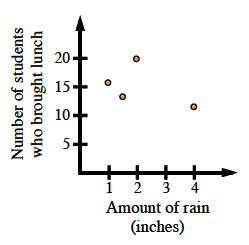### Home > MC1 > Chapter 6 > Lesson 6.2.2 > Problem6-62

6-62.

The following points represent the number of inches of rain each day and the number of students in Ms. B's class who brought their lunch:

($4$ inches, $12$ students), ($2$ inches, $20$ students), ($1$ inch, $16$ students), ($1.5$ inches, $13$ students).

1. Graph the data.

What should be the horizontal axis? The vertical axis? Make sure to create your own graph before checking the answer.2. Does the number of students who bring their lunch seem to be related to the number of inches of rain each day? Explain.

Does there seem to be any pattern to the graph? If there is more rain, do more students pack lunch?
If there is more rain, do fewer students pack lunch?